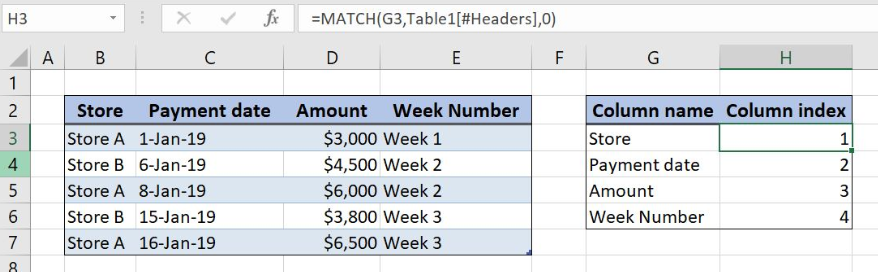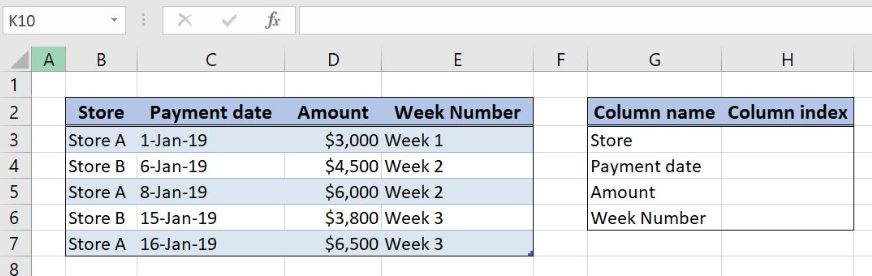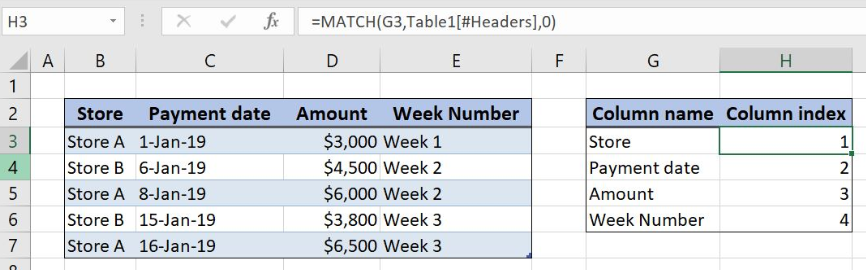Get instant live expert help with Excel or Google Sheets“My Excelchat expert helped me in less than 20 minutes, saving me what would have been 5 hours of work!”

#### Post your problem and you’ll get Expert help in seconds.

Your message must be at least 40 characters
Our professional Expert are available now. Your privacy is guaranteed.

# Get column index in Excel Table

Excel allows us to get the column number from the Excel Table using MATCH function. The MATCH function logic is the same as for the regular cell ranges. The only difference is coming from the lookup array referencing in the Excel Table. This step by step tutorial will assist all levels of Excel users to get Excel Table column index.Figure 1. How to get column index in Excel table

## Syntax of the MATCH Formula

`=MATCH(lookup_value, lookup_array, [match_type])`

The parameters of the MATCH function are:

• lookup_value – a value which we want to find in the lookup_array
• lookup_array – the array where we want to find a value
• [match_type] – a type of match. We put 0 which is an exact match.

## Setting up Our Data to Get Excel Table Column Number

Our first table consists of 4 columns: “Store” (column B), “Payment date” (column C), “Amount” (column D) and “Week Number” (column E). This table is defined as an Excel Table with the name “Table 1”.The second one has 2 columns: “Column name” (Column G) and “Column index” (Column H). The idea is to get column number from the first table based on the column name in Column G.Figure 2. Table structure for Excel Table column index

## MATCH Function to get Column Index from Table 1

We want to get column index from the first table based on the “Column name” in the Column G.

The formula looks like:

`=MATCH(G3,Table1[#Headers],0)`

The lookup_value in the MATCH function is the cell G3, the first table column name. The lookup_array is the Table 1 header, Table1[#Headers], while the match_type is 2.

To apply the MATCH function to get the Excel table column index we need to follow these steps:

• Select cell H3 and click on it
• Insert the formula: `=MATCH(G3,Table1[#Headers],0)`
• Press enter
• Drag the formula down to the other cells in the column by clicking and dragging the little “+” icon at the bottom-right of the cell.Figure 3. Get column index in Excel Table with MATCH function

MATCH function returns the relative position of the cell G3 (Column name) in the Table 1 header range. Formula result is number 1 since “Store” is the first column in Table 1.

Reference of the lookup_array for the Excel Table is different from the regular cell ranges. This array refers to the header of Table 1. We can add columns and change Table 1 and the lookup_array will automatically recognize range changes.

Most of the time, the problem you will need to solve will be more complex than a simple application of a formula or function. If you want to save hours of research and frustration, try our live Excelchat service! Our Excel Experts are available 24/7 to answer any Excel question you may have. We guarantee a connection within 30 seconds and a customized solution within 20 minutes.

### Did this post not answer your question? Get a solution from connecting with the expert.Another blog reader asked this question today on Excelchat:
Solution examplesI need help with a vlookup type formula that will take a given name and reference it against a list of names in columns D-O. then take a given date and reference it against dates in columns A10-A440, then return the cell or its contents at the intersection of those that column and row.
Solved by I. H. in 13 minsHow do I prevent Duplicate entries in multiple columns in Excel using data validation in MSExcel 2016. I would like to prevent the user from data entering a code more than once in three column ranges
Solved by B. D. in 60 minsindex and match with duplicates. I need to use another column as a reference, so my return value has two match the value of two things for it to return
Solved by E. H. in 60 minsI have a file in which I need to somehow use a conditional formatting for the same names of the data available, or if there is any other way to calculate the frequency of one particular name appearing.
Solved by K. F. in 51 minsI need a formula to compare the data in two columns and then export the mismatched data in the 3rd column
Solved by S. Q. in 20 mins## Subscribe to Excelchat.coAnother blog reader asked this question today on Excelchat: